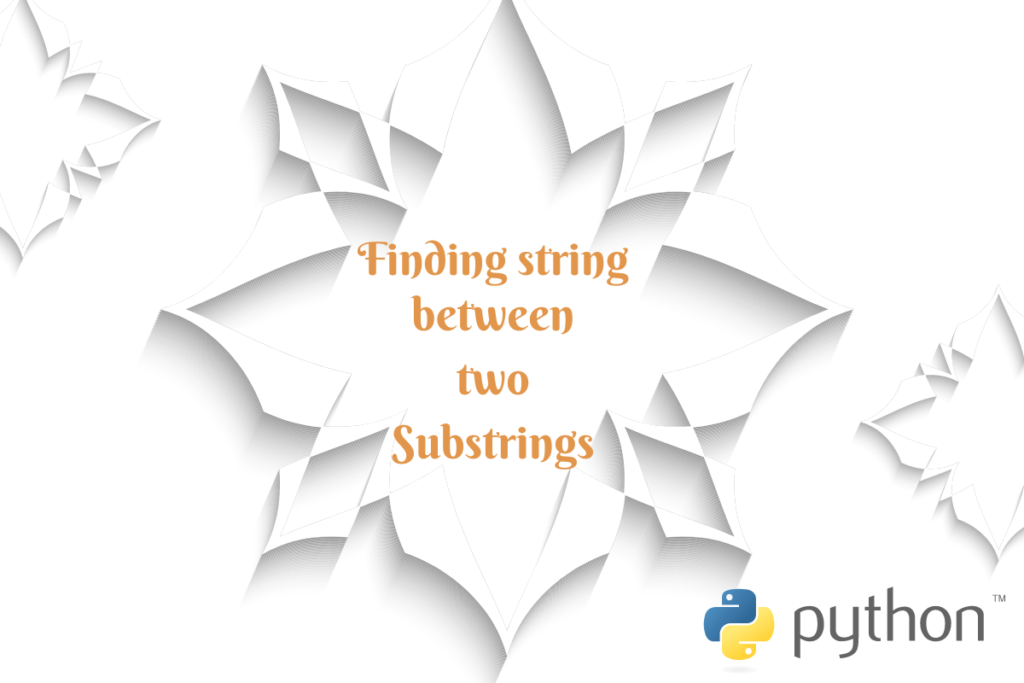# Extracting a String Between Two Substrings in PythonKeywords – they play a major role in many areas where textual data is being used. Detecting those words would pave way for the next set of actions that the program needs to pursue in machine learning applications. In this article, we shall explore one such use case where Python shall be used to extract a string between two substrings.

The keywords in this case would be the substrings and the objective is to find the string that lies between them. Following phrase shall be used for demonstration throughout this article.

```input = "Recession – Expect the unexpected when things get worse before becoming better."
```

In this tutorial, we will demonstrate four different methods to achieve this task. Let’s dive into each one of them.

• Using the index function and for loop
• Using the find function and for loop
• Using the index function with slicing
• Using the find function with slicing

## Method 1: Using the index() function and a for loop

We shall deploy a combination for both the index function and for loop to get the string between the substrings ‘when’ and ‘before’ from the input sentence. Since the for loop is being deployed let us declare a variable that could act as a marker for the substring as shown below.

```substr = " "
```

Once done it is time to construct the for loop. Within the loop we shall make use of the index function to alert the execution while it reaches the position of our target keywords ‘when’ and ‘before’. The substring variable is also incremented to factor in the iteration that shall occur when the loop is executed.

Here’s the complete code

```input = "Recession – Expect the unexpected when things get worse before becoming better."
substr = " "
for i in range(input.index("when")+len("when")+1,input.index("before")):
substr = substr + input[i]
print(substr)
```

After the above code is run, the string that lies between the target substrings is returned.

## Method 2: Using the find() function and a for loop

For those who don’t fancy putting into use the index function, there is an alternate that might be used in its place – the find function. Similar to the above technique, after declaring the input and setting the substring variable to zero, one can get started with the construction of for loop.

```input = "Recession – Expect the unexpected when things get worse before becoming better."
substr = " "
for i in range(input.find("when")+len("when")+1,input.find("before")):
substr = substr + input[i]
print(substr)
```

## Method 3: Using the index() function with slicing

Yet another variant using which one can extract the string between two substrings is the slicing technique. But, this technique does not work in silos rather than in combination with another function; to be specific the index function.

One can also try declaring the substrings against variables rather than declaring them directly in the slicing code such as those given below.

```substr1 = "when"
substr2 = "before"
```

After setting the substring variables one can right away start with the slicing operation using the code given below.

```input = "Recession – Expect the unexpected when things get worse before becoming better."
substr1 = "when"
substr2 = "before"
substr = input[input.index(substr1)+len(substr1)+1:input.index(substr2)]
```

The logic here is simple. Pull in the input and declare the substrings between which the target string lies and let the slicing do its magic.

## Method 4: Using the find() function with slicing

Similar to the above technique, one can replace the index function with the find function and deploy the same slicing to extract the target string between the substrings as shown below.

```input = "Recession – Expect the unexpected when things get worse before becoming better."
substr1 = "when"
substr2 = "before"
substr = input[input.find(substr1)+len(substr1)+1:input.find(substr2)]
```